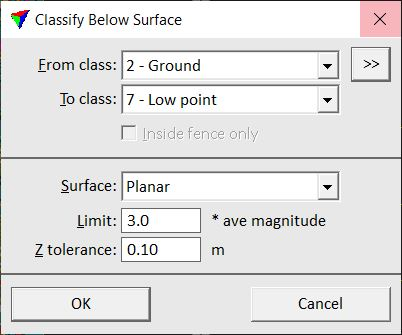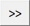# TerraScan User Guide

 Navigation: Batch Processing Reference > Classification Routines > Points Below surface

Below surface

Not Lite

Below surface routine classifies points which are lower than neighbouring points in the source class. This routine can be run, for example, after ground classification to locate points which are a bit below the true ground surface.

For each point, the routine finds up to 25 closest neighbour points in the source class. It fits a planar or curved plane to the neighbouring points and computes the average magnitude of the elevation differences between the points and the plane. If the point is more than the average magnitude multiplied by a given factor below the plane, it is classified. If the point is above the plane or less than a given tolerance value below the plane, it is not classified.Below point classificationSETTING

EFFECT

From class

Source class(es) from which points are classified.Opens the Select classes dialog which contains the list of active classes in TerraScan. You can select multiple source classes from the list that are then used in the From class field.

To class

Target class.

Inside fence only

If on, points inside a fence or selected polygon(s) are classified.

Surface

Shape of the surface that is used as reference for classifying points: Planar or Curved.

Limit

Factor for multiplication with the average magnitude of the elevation differences of neighbouring points. A point is classified, if it is more than Limit * avg magnitude below the plane fitted through the neighbour points.

Z tolerance

Maximum allowed elevation variation of a point below the fitted plane. A point within this tolerance distance is not classified.

TerraScan User Guide   26.07.2021   © 2021 Terrasolid Ltd